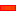This topic:

How we can normalize Payload Types with coefficients: Q, K, Cm

Reviews the effect on input-torque for different payloads types, and how we can normalize it as the coefficient, Cc

Notes on Terminology:

Payload, also named load, is the total force, or torque, referred to the cam-follower bearing.

Load imposed on a cam-mechanism is also known as the duty of the mechanism.

Capacity is the ability of the mechanism to perform the duty for a specified lifetime.

###Input Torque Coefficient

#### Input Torque Coefficient, Cc (K= 1, and Q= 0 – 1)Drive Torque Fluctuations with each Motion-Law. It is common to use the maximum value of the Input Torque Coefficient, Cc, when the inertia ratio, Q=1 Simple Harmonic Motion varies smoothly and gradually from a low positive peak to a moderate negative: this is a good characteristic. However, if this motion-law is preceded or followed by a dwell, there will be a discontinuity of acceleration at the start and end of the motion, and the dynamic response may be unacceptable.•When the Inertia Ratio is 1, the input torque of the Modified-Trapezoid varies rapidly from a high positive to a low negative value at cross-over (X=0.5): •A rapid reversal of Torque is bad because the input power source (motor) reaches a high drive torque which must rapidly to high braking torque. This might cause severe overrun if the input transmission has low inertia. Over-run means the drive speed is greater than the nominal drive speed of the cam-shaft.•If the input has a low rigidity, the drive shaft will suddenly 'unwind' to reverse its direction of 'twist'. This can cause input speed-oscillation of the cam-shaft.•When the Inertia Ratio is 1, the input torque of the Cycloidal varies more gradually than the Mod-Trap motion-law , however, not as gradually as the Mod-Sine, and it also has a higher peak value than the Mod-Sine motion-law.•When the Inertia Ratio is 1, the input torque of the Mod-Sine varies gradually from positive to negative. •It varies more gradually than, and is better than, Cycloidal. This makes the Mod-Sine very tolerant of a bad input transmission which, combined with its good dynamic response properties, is the reason for its popularity.This image shows the: Peak Values of Input-Torque Coefficient Cc against Q , Inertia Ratio, for each motion-law for constant loading (K=1).